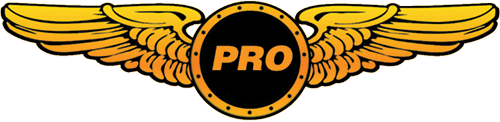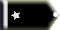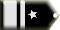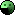Textures =[Flyguy41983 Trainee

ok so I recently downloaded a Simbuilders Airbus A340-600 and a Virgin Atlantic texture. I have tried hard to get the textures to work but all i get is the non-textured plain grey aircraft. I put the texture file into the folder but nothing shows up. How do I get the textures to work?

3 ResponsesThis should help.Tailhook Chief Captain

Flyguy41983 wrote:

Unfortunately you're not telling us which file you downloaded. Most of the still available Simu 340-600/500s are incomplete or textures only.

Here's what I've done:

http://library.avsim.net/esearch.php?DLID=71053

This file contains the model and FDE which is a must. It does NOT include a panel or sound folder - but you can copy and paste these over from your default 737 (sound) and 747 (panel) for the time being. At least you'll have a flyable aircraft now.

http://library.avsim.net/esearch.php?DLID=&Name=&FileName=vir_a346.zip&Author=&CatID=root

...just drop the folder named texture.vir into your Airbus 340-600 Lufthansa folder.

Open the aircraft.cfg with notepad, delete all of the text and replace it with this:

[fltsim.0]
title=Airbus 340-600 Lufthansa
sim=a340
model=
panel=
sound=
texture=LU
kb_checklists=
kb_reference=
atc_id=D-AIHK
atc_airline=Lufthansa
atc_flight_number=
atc_heavy=1
ui_manufacturer=Airbus
ui_type=A340-600
ui_variation=Lufthansa
visual_damage=1
description=The Airbus 340-600 is Airbus' newest production from the vernerable A340 family. This aircraft comes standard with common Airbus features all of pilots have come to respect (Fly by Wire controls, Next Generation Cockpit design, ETC.,), while maintaining a standard adapatation/familiarization environment which provides a comfortable working and learning setting for flight crew and general crew alike.

[fltsim.1]
title=Airbus 340-600 VIR
sim=a340
model=
panel=
sound=
texture=vir
kb_checklists=
kb_reference=
atc_id=
atc_airline=Virgin Atlantic
atc_flight_number=
atc_heavy=1
ui_manufacturer=Airbus
ui_type=340-600
ui_variation=Virgin Atlantic
visual_damage=1
description=The Airbus 340-600 is Airbus' newest production from the vernerable A340 family.
This aircraft comes standard with common Airbus features all of pilots have come to respect
(Fly by Wire controls, Next Generation Cockpit design, ETC.,), while maintaining a standard adapatation/familiarization environment
which provides a comfortable working and learning setting for flight crew and general crew alike.

[General]
atc_type=AIRBUS
atc_model=A340
editable=1
performance=Maximum Cruise: Mach .86\nEngines: Four (4) RR Trent 556 Turbofans\nThrust: 56,000 lbs or 20,901 KG\nMaximum Range: 7480 NM or 13,853 KM\nPax Capacity: 380 - 475 Passengers\nSimuBuild Freeware Group\nDesigner: Herve Dongmo\nFlight Dynamics: SimuBuild FDX Engineering Department\nTextureing: SimuBuild Texture Department\nFlight Deck: SimuBuild Flightdeck Engineering.\n Visit us at simubuild.cjb.net\nV1K-2

[pitot_static]
vertical_speed_time_constant=1 //Increasing this value will cause a more instantaneous reaction in the VSI

[WEIGHT_AND_BALANCE]
max_gross_weight=920000 // (pounds)
empty_weight=391000 // (pounds)

reference_datum_position=0, 0, 0 // (feet) distance from FlightSim Reference position: (1/4 chord, centerline, waterline)
empty_weight_CG_position=-2, 0, 0 // (feet) longitudinal, lateral, vertical distance from specified datum

max_number_of_stations=50

station_load.0=10620, 78, 0,0.98 //PAX SEC 1 (DEFAULT:10620)
station_load.1=18360,-0.55, 0,0.98 //PAX SEC 2 (DEFAULT:18360)
station_load.2=8100,-55.7, 0,0.98 //PAX SEC 3 (DEFAULT:8100)

station_load.4=4037, 96.03,0,-4.26 //FORE CARGO COMPARTMENT (DEFAULT: 4037)
station_load.5=3131,-69.16,0,-4.26 //AFT LARGE CARGO COMPARTMENT (DEFAULT: 3131)
station_load.6=1071,-76.96,0,-4.26 //AFT SMALL CARGO COMPARTMENT (DEFAULT: 1071)

//PILOT AND RELIEF WEIGHT
station_load.8=180,1.6,118.1,1.6 //FIRST OFFICER WEIGHT (DEFAULT: 180)

;Moments of Inertia
empty_weight_roll_MOI=15352310
empty_weight_pitch_MOI=24223159
empty_weight_yaw_MOI=39531785
empty_weight_coupled_MOI=0
;2142

[fuel]
Center1=21.3, 0.0, -7.0, 14788.0, 7.0 //Longitudinal (feet), Lateral (feet), Vertical (feet), Usable(gallons), Unusable (gallons)
Center2=-107.0, 0.0, 6.5, 2177.0, 0.0 //Longitudinal (feet), Lateral (feet), Vertical (feet), Usable(gallons), Unusable (gallons)
LeftMain=20, -50.1, -4.0, 15588.0, 9.0 //Longitudinal (feet), Lateral (feet), Vertical (feet), Usable(gallons), Unusable (gallons)
LeftAux=-2.3, -63.0, -3.0, 1680.0, 0.0 //Longitudinal (feet), Lateral (feet), Vertical (feet), Usable(gallons), Unusable (gallons)
RightMain=20, 50.1, -4.0, 15588.0, 9.0 //Longitudinal (feet), Lateral (feet), Vertical (feet), Usable(gallons), Unusable (gallons)
RightAux=-2.3, 63.0, -3.0, 1680.0, 0.0 //Longitudinal (feet), Lateral (feet), Vertical (feet), Usable(gallons), Unusable (gallons)
fuel_type=2 //Fuel type: 1 = Avgas, 2 = JetA
number_of_tank_selectors=1
electric_pump=1

[airplane_geometry]
wing_area=4729.16 //Square feet
wing_span=208.5 //Feet
wing_root_chord=45.3 //Feet
wing_dihedral=7.0 //Degrees
wing_incidence=2.0 //Degrees
wing_twist=-1.0 //Degrees
oswald_efficiency_factor=0.81 //Measure of lift effeciency of wing
wing_winglets_flag=1 //Are winglets available?
wing_pos_apex_lon=-68.2 //Feet, longitudinal distance from reference point, negative going aft
wing_pos_apex_vert=0 //Feet, vertical distance from reference point, positive going up
htail_area=1470 //Square feet
htail_span=72.8 //Feet
htail_pos_lon=-210.0 //Feet, longitudinal distance from reference point, negative going aft
htail_pos_vert=0.0 //Feet, vertical distance from reference point, positive going up
htail_incidence=0.0 //Degrees
htail_sweep=37.5 //Degrees, horizontal tail leading edge
vtail_area=1060 //Square feet
vtail_span=37.1 //Feet, tip to body
vtail_sweep=45.0 //Degrees, vertical tail leading edge
vtail_pos_lon=-198.5 //Feet, longitudinal distance from reference point, negative going aft
vtail_pos_vert=26.1 //Feet, vertical distance from reference point, positive going up
elevator_area=327 //Square feet
aileron_area=225 //Square feet
rudder_area=230 //Square feet
elevator_up_limit=30 //Degrees
elevator_down_limit=17 //Degrees
aileron_up_limit=30 //Degrees
aileron_down_limit=20 //Degrees
rudder_limit=35 //Degrees
elevator_trim_limit=16 //Degrees
spoiler_limit=35 //Degrees
spoilerons_available=1 //Spoilerons Available?
aileron_to_spoileron_gain=2 //Aileron to spoileron gain
min_ailerons_for_spoilerons=15 //Degrees
min_flaps_for_spoilerons=0 //Minimum flap handle position when spoilerons activate

[Reference Speeds]
flaps_up_stall_speed=224.0 //Knots True (KTAS)
full_flaps_stall_speed=155.0 //Knots True (KTAS)
cruise_speed=500.0 //Knots True (KTAS)
max_indicated_speed=370.671875
max_mach=0.904980

//

[flaps.0] //Inboard Trailing Edge Flaps
type=1 // 1 - tail, 2 - lead
span-outboard=0.2 // 0.0 .. 1.0
extending-time=25 // seconds
flaps-position.0=0 // degrees
flaps-position.1=0 // degrees
flaps-position.2=17.5 // degrees
flaps-position.3=22.5 // degrees
flaps-position.4=26.5 // degrees
flaps-position.5=32 // degrees
damaging-speed=269 // KIAS
blowout-speed=327 // KIAS
lift_scalar=0.38
drag_scalar=0.25
pitch_scalar=0.17
system_type=1 //Hydraulic

[flaps.1] //Outboard Trailing Edge Flaps
type=1 // 1 - tail, 2 - lead
span-outboard=0.2 // 0.0 .. 1.0
extending-time=25 // seconds
flaps-position.0=0 // degrees
flaps-position.1=0 // degrees
flaps-position.2=17.5 // degrees
flaps-position.3=22.5 // degrees
flaps-position.4=26.5 // degrees
flaps-position.5=32 // degrees
damaging-speed=269 // KIAS
blowout-speed=327 // KIAS
lift_scalar=0.38
drag_scalar=0.25
pitch_scalar=0.17
system_type=1 //Hydraulic

type=2 // 1 - tail, 2 - lead
span-outboard=0.2 // 0.0 .. 1.0
extending-time=11 // seconds
flaps-position.0=0 // degrees
flaps-position.1=21 // degrees
flaps-position.2=21 // degrees
flaps-position.3=24 // degrees
damaging-speed=260 // KIAS
blowout-speed=300 // KIAS
lift_scalar=0.33
drag_scalar=0.11
pitch_scalar=0.35
system_type=1 //Hydraulic

[flight_tuning]
cruise_lift_scalar=1.1
parasite_drag_scalar=2.1
induced_drag_scalar=1.8
elevator_effectivenes=1.0
aileron_effectiveness=0.7
rudder_effectiveness=1.0
pitch_stability=1.0
roll_stability=1.0
yaw_stability=1.0
elevator_trim_effectiveness=1.0
aileron_trim_effectiveness=1.0
rudder_trim_effectiveness=1.0

[GeneralEngineData]
engine_type=1 //0=Piston, 1=Jet, 2=None, 3=Helo-Turbine, 4=Rocket, 5=Turboprop
Engine.0=-107.5, -73.0, -6.9 //(feet) longitudinal, lateral, vertical distance from reference datum
Engine.1=-76.0, -38.9, -10.4 //(feet) longitudinal, lateral, vertical distance from reference datum
Engine.2=-76.0, 38.9, -10.4 //(feet) longitudinal, lateral, vertical distance from reference datum
Engine.3=-107.5, 73.5, -6.9 //(feet) longitudinal, lateral, vertical distance from reference datum
fuel_flow_scalar=1.05 //Scalar for fuel flow efficiency
min_throttle_limit=-0.250000

[TurbineEngineData]
fuel_flow_gain=0.002 //Gain on fuel flow
inlet_area=56.2 //Square Feet, engine nacelle inlet area
rated_N2_rpm=29920 //RPM, second stage compressor rated value
static_thrust=56000 //Lbs, max rated static thrust at Sea Level
afterburner_available=0 //Afterburner available?
reverser_available=1 //Thrust reverser available?

[jet_engine]
thrust_scalar=0.9

[autopilot]
autopilot_available=1
flight_director_available=1
default_vertical_speed=1000.0
autothrottle_available=1
autothrottle_arming_required=1
autothrottle_max_rpm=96
autothrottle_takeoff_ga=1
pitch_takeoff_ga=8;
max_pitch=10.000000
max_pitch_acceleration=1.000000
max_pitch_velocity_lo_alt=2.000000
max_pitch_velocity_hi_alt=1.500000
max_pitch_velocity_lo_alt_breakpoint=20000.000000
max_pitch_velocity_hi_alt_breakpoint=28000.000000
max_bank=25.000000
max_bank_acceleration=1.800000
max_bank_velocity=3.000000
max_throttle_rate=0.100000
nav_proportional_control=9.000000
nav_integrator_control=0.250000
nav_derivative_control=0.000000
nav_integrator_boundary=2.500000
nav_derivative_boundary=0.000000
gs_proportional_control=9.520000
gs_integrator_control=0.260000
gs_derivative_control=0.000000
gs_integrator_boundary=0.700000
gs_derivative_boundary=0.000000
yaw_damper_gain=0.000000

[contact_points]

//0 Class <0=none,1=wheel, 2=scrape, 3=float>
//1 Longitudinal Position (feet)
//2 Lateral Position (feet)
//3 Vertical Position (feet)
//4 Impact Damage Threshold (Feet Per Minute)
//5 Brake Map (0=None, 1=Left, 2=Right)
//7 Steer Angle (degrees)
//8 Static Compression (feet) (0 if rigid)
//9 Max/Static Compression Ratio
//10 Damping Ratio (0=Undamped, 1=Critically Damped)
//11 Extension Time (seconds)
//12 Retraction Time (seconds)
//13 Sound Type
//14 Airspeed limit for retraction (KIAS)
//15 Airspeed that gear gets damage at (KIAS)

//Main and Nose Gear Contact Points
point.0=1, 102.5, 0.0, -17.1, 1500.0, 0, 1.584, 85, 0.6, 3, 2.100, 9.0, 8.0, 0, 220, 262
point.1=1, -9, 0.0, -18.90, 2000.0, 0, 1.837, 0, 1, 1, 0.900, 11.0, 9.0, 2, 220, 262
point.2=1, -9, -17.536, -18.90, 2000.0, 1, 1.837, 0, 1, 1, 0.900, 11.0, 9.0, 3, 220, 262
point.3=1, -9, 17.536, -18.90, 2000.0, 2, 1.837, 0, 1, 1, 0.900, 11.0, 9.0, 3, 220, 262

//Tail Scrape Points
point.4=2, -72.178, 0.0, -9.51, 430.0, 0, 0.0, 0.0, 0.0, 0.0, 0.000, 0.0, 0.0, 5, 0, 0 //Crest
point.5=2, -131.23, 0.0, 6.8, 430.0, 0, 0.0, 0.0, 0.0, 0.0, 0.000, 0.0, 0.0, 6, 0, 0 //Peak

//Nose Scrape Points
point.6=2, 112.762, 0.0, 7.217, 430.0, 0, 0.0, 0.0, 0.0, 0.0, 0.000, 0.0, 0.0, 9, 0, 0 //Break for NG Door

//Fairing Scrape Points
point.7=2, 0.75, 0.0, 10.465, 430.0, 0, 0.0, 0.0, 0.0, 0.0, 0.000, 0.0, 0.0, 4, 0, 0 //Center Faring Bottom

//Wing Scrape Points
point.8=2, 40.48, -103.83, 4.845, 430.0, 0, 0.0, 0.0, 0.0, 0.0, 0.000, 0.0, 0.0, 4, 0, 0 //Left Wing
point.9=2, 40.48, 103.83, 4.845, 430.0, 0, 0.0, 0.0, 0.0, 0.0, 0.000, 0.0, 0.0, 4, 0, 0 //Right Wing

static_pitch=-0.2
static_cg_height=16.7
gear_system_type=0

[Views]
eyepoint=42.00, -7.30, 3.00 //Pilots View

[electrical]
autopilot=0, 5 , 17.0
avionics_bus=0, 10, 17.0
avionics=1, 5 , 17.0
gear_warning=0, 2 , 17.0
fuel_pump=0, 5 , 17.0
light_nav=0, 5 , 17.0
light_beacon=0, 5 , 17.0
light_landing=0, 5 , 17.0
light_taxi=0, 5 , 17.0
light_strobe=0, 5 , 17.0
light_panel=0, 5 , 17.0

// Radio Type=availiable, standby frequency, has glide slope
Audio.1=1
Com.1=1, 0
Com.2=1, 0
Nav.1=1, 0, 1
Nav.2=1, 0, 0
Transponder.1=1
Marker.1=1

[LIGHTS]
//Types: 1=beacon, 2=strobe, 3=navigation, 4=cockpit, 5=landing
light.0 =1, 18.207, 0, 9.4, fx_beaconb,
light.1 =1, 18.225, 0, -10.8, fx_beaconh,
light.2 =3, -123.839, -0.151, 6.819, fx_navwhih ,
light.3 =2, -123.839, 0.151, 6.819, fx_strobeh ,
light.4 =5, 18, 27.464, -4, A340_wingscan,
light.5=5, -1.933, 60.827, -0.368, A340_wingscan,
light.6=5, 18, -27.464, -4, A340_wingscan,
light.7=5, -1.933, -60.827, -0.368, A340_wingscan,
light.8 =4, 117.038, 0, 2.812, A340_cockpit,

[direction_indicators]
//1 Type: 0=None, 1=Vacuum Gyro, 2=Electric Gyro, 3=Electro-Mag Slaved Compass, 4=Slaved to another
//2 Indicator number of slaving indicator if Type=4
direction_indicator.0=3,0

[keyboard_response]
elevator=150, 250
aileron=150, 250
rudder=150, 250

[EFFECTS]
wake=fx_wake
water=fx_spray
dirt=fx_tchdrt
concrete=fx_sparks
touchdown=fx_tchdwn, 1

[exits]
number_of_exits=2
exit_rate.0=0.4 ;Percent per second
exit_rate.1=0.4 ;Percent per second
;1660

[forcefeedback]
gear_bump_nose_magnitude=3000 ; 0 - 10000
gear_bump_nose_direction=18000 ; 0 - 35999 degrees
gear_bump_nose_duration=250000 ; in microseconds
gear_bump_left_magnitude=2700 ; 0 - 10000
gear_bump_left_direction=35500 ; 0 - 35999 degrees
gear_bump_left_duration=250000 ; in microseconds
gear_bump_right_magnitude=2700 ; 0 - 10000
gear_bump_right_direction=00500 ; 0 - 35999 degrees
gear_bump_right_duration=250000 ; in microseconds
ground_bumps_magnitude1=1300 ; 0 - 10000
ground_bumps_angle1=08900 ; 0 - 35999 degrees
ground_bumps_intercept1=3.0
ground_bumps_slope1=0.20
ground_bumps_magnitude2=200 ; 0 - 10000
ground_bumps_angle2=09100 ; 0 - 35999 degrees
ground_bumps_intercept2=1.075
ground_bumps_slope2=0.035
crash_magnitude1=10000 ; 0 - 10000
crash_direction1=01000 ; 0 - 35999 degrees
crash_magnitude2=10000 ; 0 - 10000
crash_direction2=9000 ; 0 - 35999 degrees
crash_period2=75000 ; in microseconds
crash_duration2=2500000 ; in microseconds
[brakes]
toe_brakes_scale=1.241798
parking_brake=1
[gear_warning_system]
gear_warning_available=1
pct_throttle_limit=0.099976
flap_limit_power=25.496622
flap_limit_idle=5.042029
[hydraulic_system]
electric_pumps=0
engine_map=1,1,1,1
normal_pressure=3000.000000
[stall_warning]
type=1
[attitude_indicators]
attitude_indicator.0=1
[turn_indicators]
turn_indicator.0=1,0

The aircraft will show up under Airbus in your Select an Aircraft Menu. Any problems, ask 😎Flyguy41983 Trainee

Thank you all so much.
I finally got the textures to work!!

All times are GMT Page 1 of 1